# AP Physics B : Magnetism

## Example Questions

### Example Question #3 : Magnetic Force

A proton traveling at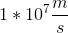in a horizontal plane passes through an opening into a mass spectrometer with a uniform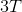magnetic field directed upward. The particle then moves in a circular path throughand crashes into the wall of the spectrometer adjacent to the entrance opening. How far down from the entrance is the proton when it crashes into the wall?

The proton’s mass is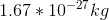and its electric charge is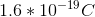.

Possible Answers: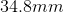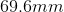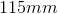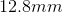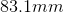Correct answer:Explanation: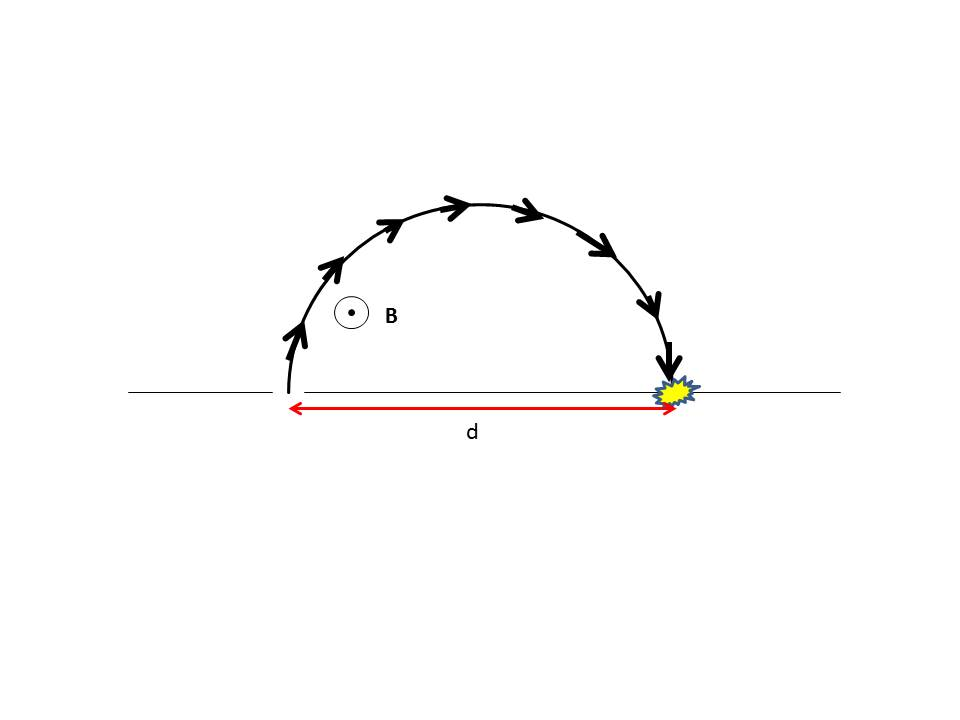A charged particle moving through a perpendicular magnetic field feels a Lorentz force equal to the formula: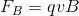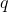is the charge,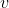is the particle speed, andis the magnetic field strength. This force is always directed perpendicular to the particle’s direction of travel at that moment, and thus acts as a centripetal force. This force is also given by the equation: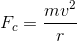We can set these two equations equal to one another, allowing us to solve for the radius of the arc.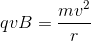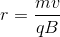Once the particle travels through a semicircle, it is laterally one diameter in distance from where is started (i.e. twice the radius of the circle).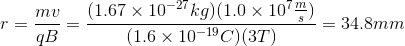Twice this value is the lateral offset of its crash point from the entrance: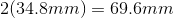### All AP Physics B Resources